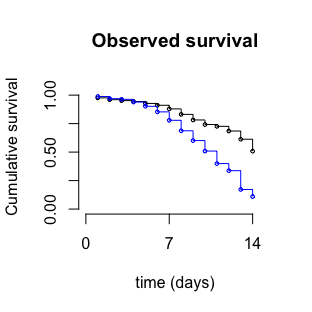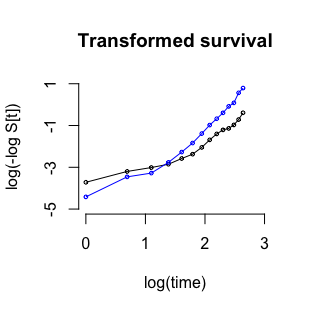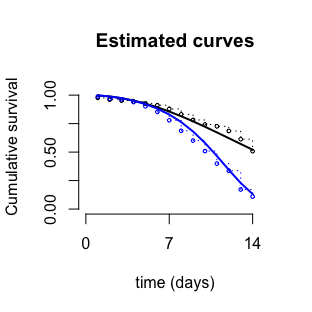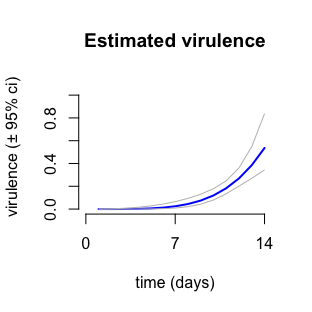# Starting values

library(anovir)

### Introduction

Maximum likelihood estimation techniques used to solve likelihood problems require initial values for the parameters to be estimated. The estimation process starts from these values and progressively adjusts them iteratively until the fit between the likelihood model and the observed data does not improve beyond a threshold value. The process of convergence to this solution is enhanced and a solution more likely to be found when the starting values are close to the 'true' values.

This vignette illustrates how starting values can be estimated by linear or non-linear regression of observed cumulative survival data.

### Linear regression of transformed cumulative survival data

The cumulative survival functions of some probability distributions transform into linear functions of time.

For example the cumulative survival function, S(t), of the Weibull distribution,

$\begin{equation} S(t) = \exp \left( - \exp \left[ \frac{\log t - a}{b} \right] \right) \end{equation}$

is a linear function of log(t) following a complementary log-log transformation,

$\begin{equation} \log \left( - \log \left[ S(t) \right] \right) = \frac{1}{b} \log t - \frac{a}{b} \end{equation}$

where a and b are the location and scale parameters, respectively.

An advantage of this linearisation is that observed cumulative survival data given a complementary log-log transformation and plotted against log(t) will be approximately linear, if they follow the Weibull distribution.

When this is the case, the coefficients for the slope and intercept of a linear regression can be used to estimate the location and scale parameters of the distribution.

This approach is particularly suited to data from uninfected or control treatments, where a single survival function is assumed to describe the pattern of survival in the treatment as a whole.

This is not the case for infected treatments, where the relative survival approach assumes the observed patterns of host survival arise as the product of cumulative survival functions for background mortality and mortality due to infection [1–4].

The contribution of background mortality, SUNINF(t), to the cumulative survival observed in an infected treatment, SOBS.INF(t), can be removed by calculating their relative survival, SREL(t),

$\begin{equation} S_{REL}(t) = \frac{S_{OBS.INF}(t)}{S_{UNINF}(t)} = \frac{S_{INF}(t) \cdot S_{UNINF}(t)}{S_{UNINF}(t)} = S_{INF}(t) \\ \end{equation}$

which equals host survival due only to the effects of infection, SINF(t).

The latter can be transformed and plotted against time for evidence of a linear relationship. When this is the case, linear regression allows the location and scale parameters of the distribution describing mortality due to infection to be estimated.

The product of two Weibull cumulative survival functions is a cumulative survival function that also follows the Weibull distribution. Hence from the relationship, SOBS.INF(t) = SUNINF(t) x SINF(t), it follows that if the observed cumulative survival in an infected treatment and that in a matching uninfected treatment are both approximately linear when given a complementary log-log transformation and plotted against log(t), the unobserved pattern of mortality due to infection is also likely to follow the Weibull distribution.

The cumulative survival functions for the Gumbel and Fréchet distribution can also transformed into linear functions of time; see Probability distributions for details.

### Non-linear regression of cumulative survival data

The nonlinear least squares, nls, function from the package 'stats' of R  can be used to directly estimate the location and scale parameters from untransformed cumulative survival data.

As for linear regression, this approach is more suited to estimating location and scale parameters of a single survival function, rather those for two functions at the same time.

In this case, the estimation of parameters for mortality due to infection can be estimated from the cumulative relative survival of infected hosts. Alternatively the observed cumulative survival in an infected treatment can be estimated as the product of two cumulative survival functions, where the values of the location and scale parameters for background mortality are given fixed values based on a non-linear regression of data in the matching uninfected or control population (see below).

### Limitations to regression of cumulative survival data

The location and scale parameters estimated by the linear or non-linear regression of cumulative survival data should be treated as approximate.

Two reasons for this are because both approaches use summary data, rather than data from individual hosts, and the degrees of freedom on which these regressions are based depend on the number of sampling intervals in the dataset, rather than the number of individuals dying or censored in each interval.

The negative log-likelihood (nll) models in this package also rely upon regression. However the maximum likelihood approach to estimating parameters uses data from individual hosts and explictly takes into account the frequencies of individuals dying or censored in each sampling interval.

### Example

The following example uses a subset of data from the study by Blanford et al  and made available here by kind permission of Matthew Thomas. The data are for the uninfected and infected treatments, cont and Bb06, respectively, from the third block of the experiment. These data were used to calculate survival in both treatments over time and stored in data01

The first figure below shows the pattern of survival in the uninfected cont treatment (black) and in the infected treatment Bb06 (blue).

The second figure shows the survival data following a complementary log-log transformation plotted against log(time). The approximately linear plots for both treatments indicate the Weibull distribution was suitable for describing both background mortality and mortality due to infection.#### Linear regressions

The observed cumulative survival of uninfected hosts was given a complementary log-log transformation and used in a linear regression against log(time);

lr_clogSuninf <- lm(data01$clogSuninf ~ data01$log_time, data = data01)
coef(lr_clogSuninf)
#>     (Intercept) data01$log_time #> -4.228407 1.266274 df.residual(lr_clogSuninf) #>  12 The values estimated for the slope and intercept of this regression were used to estimate the parameters for the Weibull distribution describing background mortality; b = 1/1.266 = 0.79 a = 4.228 * 0.79 = 3.339 The relative survival of infected hosts was calculated, given a complementary log-log transformation, and used in a linear regression against log(time); lr_clogSrel <- lm(data01$clogSrel ~ data01$log_time, data = data01) coef(lr_clogSrel) #> (Intercept) data01$log_time
#>      -10.507528        4.177483
df.residual(lr_clogSrel)
#>  9

The values estimated for the slope and intercept of this regression were used to calculate the parameters for the Weibull distribution describing mortality due to infection;

b = 1/4.177 = 0.239

a = 10.508 * 0.239 = 2.515

There were 12 residual degrees of freedom for the regression of uninfected host data, but only 9 for infected hosts. This difference arises because a few more uninfected hosts died than infected hosts at the beginning of the experiment. This lead to the relative survival of infected hosts being calculated as greater than one (> 1) for three sampling intervals. A complementary log-log transformation cannot be calculated for these estimates due to a negative value of -log(x) when x > 1.

#### Non-linear regressions

The nls function used a formula for the obsersved cumulative survival of uninfected hosts as a function of the Weibull survival function to estimate the location, a1, and scale, b1 parameters for background mortality;

     nlr01 <- nls(Suninf ~ exp(-exp((log(time) - a1) / b1)),
data = data01,
start = list(a1 = 2, b1 = 1)
)
coef(nlr01)
#>        a1        b1
#> 2.8877729 0.4744913
df.residual(nlr01)
#>  12

A second nls function used a formula for the observed cumulative survival of infected hosts as a function of the product of two Weibull survival functions for background mortality and mortality due to infection. The values estimated above for the location and scale parameters for background mortality were substituted into the survival function for background mortality, leaving only the location and scale parameters for mortality due to infection, a2 and b2, respectively, to be estimated;

      nlr02 <- nls(Sobsinf ~ exp(-exp((log(time) - 2.888) / 0.474)) *
exp(-exp((log(time) - a2) / b2)),
data = data01,
start = list(a2 = 2, b2 = 1)
)
coef(nlr02)
#>        a2        b2
#> 2.5419579 0.2633441
df.residual(nlr02)
#>  12

No degrees of freedom were lost in the second regression due to incompatable data transformation.

#### Maximum likelihood estimation

The data above were also estimated by maximum likelihood using a combination of nll_basic from this package and mle2 of the package bbmle .

The first step involve a 'prep function' which provided the information needed for nll_basic to calculate a negative log-likelihood given the values of a1, b1, a2, b2.

The 'prep function' was then sent to mle2 from the package bbmle for maximum likelihood estimation, using the values estimated by the linear regression of transformed cumulative survival data as staring values;

  head(subset_data_bl3, 3)
#>     block treatment replicate_cage day censor d inf t fq
#> 450     3      cont              1   1      0 1   0 1  0
#> 451     3      cont              1   2      0 1   0 2  0
#> 452     3      cont              1   3      0 1   0 3  0
  # step #1: prep-function for maximum likelihood estimation
mle01_prep_function <- function(a1, b1, a2, b2){
nll_basic(
a1, b1, a2, b2,
data = subset_data_bl3,
time = day,
censor = censor,
infected_treatment = inf,
d1 = 'Weibull', d2 = 'Weibull'
)}

# step #2: start values from linear regression of transformed data
mle01 <- mle2(mle01_prep_function,
start = list(a1 = 3.339, b1 = 0.790, a2 = 2.515, b2 = 0.239)
)
coef(mle01)
#>        a1        b1        a2        b2
#> 2.8451290 0.4828339 2.5807774 0.1831184

#### Compare models

One way to compare the results of the above models is to take their estimates and use them as starting values for the 'prep function' to be sent to mle2 using the option eval.only = TRUE; this will return the likelihood calculated by nll_basic given the starting values;

   # linear regression estimates
LR <- mle2(mle01_prep_function,
start = list(a1 = 3.339, b1 = 0.790, a2 = 2.515, b2 = 0.239),
eval.only = TRUE
)

# non-linear regression estimates
NLR <- mle2(mle01_prep_function,
start = list(a1 = 2.888, b1 = 0.474, a2 = 2.542, b2 = 0.266),
eval.only = TRUE
)

# maximum likelihood estimates
MLE <- mle2(mle01_prep_function,
start = list(a1 = 2.845, b1 = 0.483, a2 = 2.581, b2 = 0.183),
eval.only = TRUE
)

# compare models by Akaike's Information Criteria, adjusted for small sample sizes
AICc(LR, NLR, MLE, nobs = 287)
#>       AICc df
#> 1 1331.450  4
#> 2 1307.543  4
#> 3 1301.286  4

According to Akaike's information criterion corrected for small sample size, AICc , the maximum likelihood model provided the best description of the data.

These estimates were used to generate the estimated cumulative survival of uninfected and infected hosts, along with the pathogen's estimated virulence.

The conf_ints_virulence function of this package was also used to calculate approximate 95% confidence intervals for the estimated virulence of the pathogen based on the variance and covariance of estimates of a2 and b2 generated by mle2.

  coef(mle01) ; vcov(mle01)
#>        a1        b1        a2        b2
#> 2.8451290 0.4828339 2.5807774 0.1831184
#>               a1            b1            a2            b2
#> a1  0.0047702128  0.0015422555 -0.0009300482  0.0007352816
#> b1  0.0015422555  0.0018532486 -0.0001779336 -0.0001257280
#> a2 -0.0009300482 -0.0001779336  0.0008196247 -0.0003118982
#> b2  0.0007352816 -0.0001257280 -0.0003118982  0.0010005684

ci_matrix01 <- conf_ints_virulence(
a2 = 2.5807774,
b2 = 0.1831184,
var_a2 = 0.0008196247,
var_b2 = 0.0010005684,
cov_a2b2 = -0.0003118982,
d2 = "Weibull", tmax = 14)